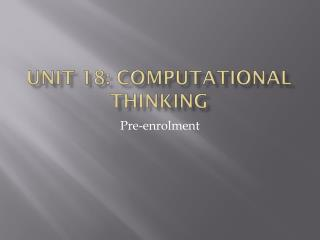DownloadDownload PresentationUnit 18: Computational Thinking

# Unit 18: Computational Thinking

Download Presentation## Unit 18: Computational Thinking

- - - - - - - - - - - - - - - - - - - - - - - - - - - E N D - - - - - - - - - - - - - - - - - - - - - - - - - - -
##### Presentation Transcript

1. Unit 18: Computational Thinking Pre-enrolment

2. Unit introduction • Computers influence much of the world around us • used to solve a range of problems, • generating the visual effects in films • landing the Curiosity Rover (unmanned robot) on Mars. • use mathematics and logic.

3. Numbers – an invention • No problem, • I have food, clothing

4. More than one… • Still not a problem, • just give them names

5. Lots more than one • Now need a system

6. Egyptians

7. Decimal system • Introduces a zero • Invented by independently by: • Babylonians, • Mayans • Indians • Uses place value • The value of a symbol depends on where it appears in the number: eg • 33 • 333 • 3333 etc

8. Decimal system • 10 symbols • 0 – 9 • Click over to new place value after 9

9. Other systems • Binary • Base 2 • Uses 1 & 0 • Hexadecimal • Base 16 • Uses additional symbols (A,B,C,D,E,F)

10. Binary • Basis of electrical data transmission • 0 = no current flowing • 1 = current flowing Decimal Binary

11. Task • 7, 5, 102 • 11, 1011, 10001100

12. three types of computational thinking skills • Logical thinking • Algorithmic thinking • Optimal thinking

13. Logical thinking • Work out how logical thinking is applied to the following games/puzzles: • Sudoku • Minesweeper

14. Sudoku • What are the ‘rules’? • Using the rules, what is this number? (There is only one possibility).

15. Minesweeper • The numbers indicate how many of the 8 surrounding squares have ‘mines’. • There are 10 mines here, how many can you • place from this start?

16. Finished game • 4 of the mines could be placed From the original screen.

17. Logical thinking • Don’t need to know • number bonds • Multiplication tables • Complex maths • Just use the available information • Don’t guess!

18. Algorithmic thinking • A bit like logical thinking • uses a predefined set of instructions to solve problems • E.g. • strategy games • Chess

19. Algorithmic thinking • Don’t need to know • number bonds • Multiplication tables • Complex maths • Just follow the instructions

20. Optimal thinking • refining one of many possible solutions to a problem to make it more efficient • E.g. • Rubik’s Cube • http://www.rubiks.com/solving-center/solve_rubiks_3x3_cube.php • Towers of Hanoi

21. Towers of Hanoi • http://www.mathsisfun.com/games/towerofhanoi.html • Give it a go – what is your best score? • What if there were 4 pegs?

22. Boolean Algebra • What does this mean?

23. Boolean logic Boolean algebra deals with the values 0 and 1 or the values false and true ‘the algebra of truth’ It is ideally suited for use in a computer: WHY? Operations using just 0 and 1 can be easily replicated using simple electronic circuits AND OR NOT XOR

24. Truth Tables • Boolean Operations are represented in Truth tables • Input (s) • Input A • Input B • Output • The operations are carried out by electronic ‘gates’

25. The simplest function - NOT The NOT gate takes an input and reverses it. also known as an inverter If the input is 1, the output is 0 If the input is 0, the output is 1 (very useful for 2s complement when we flip the bits) Symbol (used on circuit diagrams) Truth table

26. Task Find symbols and meanings for: • AND • OR • NOT • XOR Can you also write a truth table for each?

27. AND A 1 is required on both inputs to give a 1 at the output Only returns a high value if both inputs are high. Symbol Truth table

28. OR A 1 on either input gives a 1 at the output. Gives a high output if at least one of the inputs is high Symbol Truth table

29. Exclusive OR (EXOR XOR) You only get a 1 at the output when the two inputs are different Returns a high output if either (but not both) of the inputs is high. Symbol Truth table

30. Other gates • There are other gates:

31. How do Boolean operations help? • Searches: • Help to refine searches: • Find ‘this term’ AND ‘this term’ • Find either ‘this term’ or ‘this term’ (or both) • Find ‘this term’ but not ‘this term’ • Etc

32. Programming • Can be used with conditional statements: • E.g. ‘if’ statements

33. Addition So how can we use logic gates to add up? Need to know the rules of binary arithmetic: 0 + 0 = 0 0 + 1 = 1 1 + 0 = 1 1 + 1 = 0 and carry 1